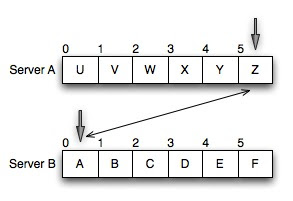## Thursday, August 12, 2010

### Distributed Sorting

So you've got a pair of servers (I'll call them servers A & B) and each of them has an array of strings in random order. You are to find a way to sort the strings such that if you lined the arrays up server A first, server B second, this combined array of strings would be sorted. That is to say: all the strings are sorted and the higher strings are all on server A and the lower strings are all on server B. These arrays take up most of the storage on the servers and your network connectivity between servers is mediocre.

How do you accomplish this with the lowest network traffic?

The naive solution is pretty simple given these constraints and looks like this:

First, sort all of the strings on each server locally.
Then compare the last string on A to the first string on B
If A[last] > B[first], swap them, then resort the strings locally on each server.
Otherwise, you are done.So what is the cost (worst case where n is the number of keys per server):

If you have to compare every single key, you'll make 2n comparisons.
And so the cost will be 2n * the size of the keys in network traffic + a small amount of overhead to open and close the connection if we use http or similar.

Great! So lets say you have a million ten character strings on each server. How long will this take? Twenty megabytes in a fast data center? A few seconds at most, totally reasonable.

And then things start to get interesting.

So... now we should consider the speed of the whole system, data transfer _and_ local sorting. A smart candidate will have already thought this far ahead, if they are accustomed to thinking about everything in terms of big O. But then it is easy to fall into the trap of thinking that network speed is so much slower than things that happen in memory that we can forget big O in situations like this. We'll see that we can't.

So, ok, we've got a good sorting algorithm O(n ln n) or maybe even O(k n) if you take advantage of the shortness of the strings to be sorted. Great, so we do that. Then, in the worst case we have to move all the strings to different servers, so that is n. AND we're resorting the strings again which should cost O(n) with each string we move across so we're looking at O(n^2).

So what does n^2 mean here? Well if we have fast memory, we can get each 10 char key out of memory in around 100 ns. So lets say (10^6)^2 * 100 ns. If I'm doing my math right here, that comes out to over 27 hours. So we spend a few seconds streaming data across the wire because we managed to minimize network traffic but we spend 27 hours resorting arrays of strings.

At this point, the fix should be pretty obvious. Move all the strings that you need to move, then sort them. But how do we know which ones to sort?

Well here is the trick, obvious to some, not obvious to others: for every string on server B that is lower in value than the lowest string on server A, we can move that string from B to A, and at the same time move the lowest string from A to B.

Think of the keys as arrays on both servers. Set a pointer at the end of the array for the first server (ie: the highest key on the lowest server) and a pointer at the start of the array on the second server (ie: the lowest key on the highest server). As long as the key at the pointer on the first server is greater than the key at the pointer on the second server, we need to swap the keys. Here is a worst case example (with single letter keys for simplicity):After each swap, we move the pointers (the first server's pointer moves left, the second server's pointer moves right) and again compare the keys under the pointers.Again, as long as the key at the pointer on the first server is greater than the key at the pointer on the second server, we need to swap the keys.When we're done, we'll again have an unsorted array of keys on each server, but we can guarantee that all the right keys will be on the right servers. From here we can just resort the servers again, and we'll be done.

Take home lesson:
1. Even though moving data around a datacenter takes a while (20 ms to send a couple k around a data center) moving data around in memory still costs some time: 1/2 a ns for L1 cache kits, 100 ns to hit main memory. Yes, orders of magnitude difference, but:
1. O(n^2) is almost always worse than O( n ln n) even when the operations described by n^2 are much, much faster.